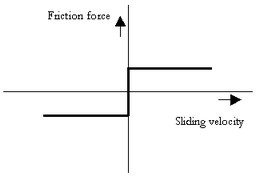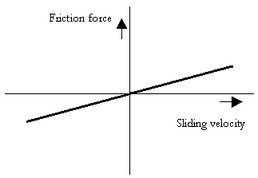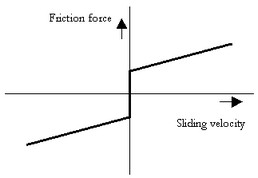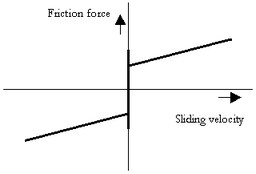﻿ 20-sim webhelp > Library > Iconic Diagrams > Mechanical > Translation > Components > FrictionSimple

# FrictionSimple

Navigation:  Library > Iconic Diagrams > Mechanical > Translation > Components >

# FrictionSimple## Library

Iconic Diagrams\Mechanical\Translation\Components

## Use

Domains: Continuous. Size: 1-D. Kind: Iconic Diagrams (Translation).

C

V

CV

SCVS

LuGre

## Use

Domains: Continuous. Size: 1-D. Kind: Iconic Diagrams (Translation).

## Introduction

These models represents friction with the fixed world. The amount of friction does not depend on the normal force but determined by parameters directly. The models have only one initial port p defined. Because any number of connections can be made, successive ports are named p1, p2, p3 etc. 20-sim will automatically create equations such that the resulting force p.F is equal to the sum of the forces of all connected ports p1 .. pn. The velocities of all connected ports are equal to p.v.

p.F = sum(p1.F, p2.F, ....)

p.v = p1.v = p2.v = ....

## Description - C

This model represents friction with the fixed world described as coulomb friction:

p.F = Fc*tanh(slope*p.v);

Fc: the coulomb friction

slope: the steepness of the coulomb and static friction curve.## Interface - C

 Ports Description p[any] Any number of connections can be made (Translation). Causality Fixed force out Parameters Fc slope Coulomb friction [N] Steepness of Coulomb friction curve [s/m]

## Description - V

This model represents friction with the fixed world described as viscous friction:

p.F = d*p.v;

d: the viscous friction or damping## Interface - V

 Ports Description p[any] Any number of connections can be made (Translation). Causality Fixed force out Parameters d Viscous friction or damping [N.s/m]

## Description - CV

This model represents friction with the fixed world described as coulomb plus viscous friction:

p.F = Fc*tanh( slope*p.v ) + d*p.v;

d: the viscous friction or damping

Fc: the coulomb friction

slope: the steepness of the coulomb and static friction curve.## Interface - CV

 Ports Description p[any] Any number of connections can be made (Translation) Causality Fixed force out Parameters Fc d slope Coulomb friction [N] Viscous friction or damping [N.s/m] Steepness of Coulomb friction curve [s/m]

## Description - SCVS

This model represents friction with the fixed world described as static plus coulomb plus viscous plus Stribeck friction:

p.F = (( Fc + (Fst*abs(tanh( slope*p.v )) - Fc) * exp( -((p.v / v_st)^2 )) ) *  sign(p.v) + d * p.v);

Fst: the static friction

d: the viscous friction or damping

Fc: the coulomb friction

slope: the steepness of the coulomb and static friction curve.

v_st: the characteristic Stribeck velocity.## Interface - SCVS

 Ports Description p[any] Any number of connections can be made (Translation). Causality Fixed force out Parameters Fc Fst d v_st slope Coulomb friction [N] Static friction [N] Viscous friction or damping [N.s/m] Characteristic Stribeck velocity [m/s] Steepness of Coulomb friction curve [s/m]

## Description - LuGre

This model represents friction with the fixed world described by the LuGre friction model:

p.F = f_lg(p.v);

f_lg: the LuGre friction model

## Interface - LuGre

Ports

Description

p[any]

Any number of connections can be made (Translation).

Causality

Fixed force out

## Parameters

Fc

Fst

d

v_st

k

Coulomb friction [N]

Static friction [N]

Viscous friction or damping [N.s/m]

Characteristic Stribeck velocity [m/s]

Stiffness at zero speed [N/m]